Next: Dayhoff Scores and Evolutionary Up: Introduction Previous: Introduction

## Model of Evolution

The model we consider here is a Markovian model of evolution , which assumes that amino acids mutate independently of each other, with probabilities which depend only on the amino acids and on the amount of evolution. In mathematical terms we can describe the model with mutation matrices: a mutation matrix, denoted by M, describes the probabilities of amino acid mutations for a given period of evolution.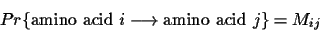Amino acids appear in nature with different frequencies. These frequencies are denoted by fi and correspond to the steady state of the Markov process defined by the matrix M, that is, the vector f is any of the columns of(M multiplied by itself an infinite number of times) or the eigenvector of M whose corresponding eigenvalue is 1 (M f = f). When we find a mutation in aligned sequences, we cannot distinguish which one mutated into which, or whether a third amino acid mutated into them. This implies a simple relation for the entries of M: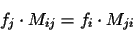M describes mutations over a given period of evolution. We must quantify this amount of change in a mathematically meaningful way. Dayhoff et. al. introduced the term PAM (point accepted mutation) unit.

Definition 1.1   A 1-PAM unit is the amount of evolution which will change, on average,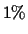of the amino acids. In mathematical terms, this is expressed as a matrix M such that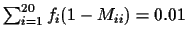, where fi is the frequency of the ith amino acid.

If we have a probability vector p, the product M p gives the probability vector after an evolution equivalent to a 1-PAM unit. After k units of evolution (a k-PAM evolution), a frequency vector p will be changed into the frequency vector Mk p. There are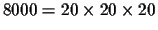possible events where an amino acid X mutates in one branch of evolution to amino acid A and to B in another branch. The sum of all these probabilities must be 1.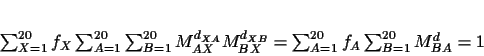(1)

where d = dXA + dXB. The above left hand side can be summed in X and we can express the probabilities of each event in terms of the children A and B (present day) and the total amount of evolution between A and B.Next: Dayhoff Scores and Evolutionary Up: Introduction Previous: Introduction
Chantal Korostensky
1999-07-14